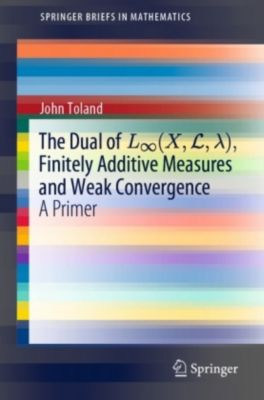# The Dual of L (X,L, ), Finitely Additive Measures and Weak Convergence

A Primer (Sprache: Englisch)Merken
Teilen
Merken
Teilen
In measure theory, a familiar representation theorem due to F. Riesz identifies the dual space L p (X,L, ) with L q (X,L, ), where 1/p+1/q=1, as long as 1 p< . However, L (X,L, ) cannot be similarly described, and is instead represented as a class of...
lieferbar
versandkostenfrei

Bestellnummer: 130247075

BuchFr. 59.00
• Kauf auf Rechnung
• Kostenlose Rücksendung
lieferbar
versandkostenfrei

Bestellnummer: 130247075

BuchFr. 59.00
In measure theory, a familiar representation theorem due to F. Riesz identifies the dual space L p (X,L, ) with L q (X,L, ), where 1/p+1/q=1, as long as 1 p< . However, L (X,L, ) cannot be similarly described, and is instead represented as a class of...

## 0 Gebrauchte Artikel zu „The Dual of L (X,L, ), Finitely Additive Measures and Weak Convergence“

ZustandPreisPortoZahlungVerkäuferRating
• Kauf auf Rechnung
• Kostenlose Rücksendung